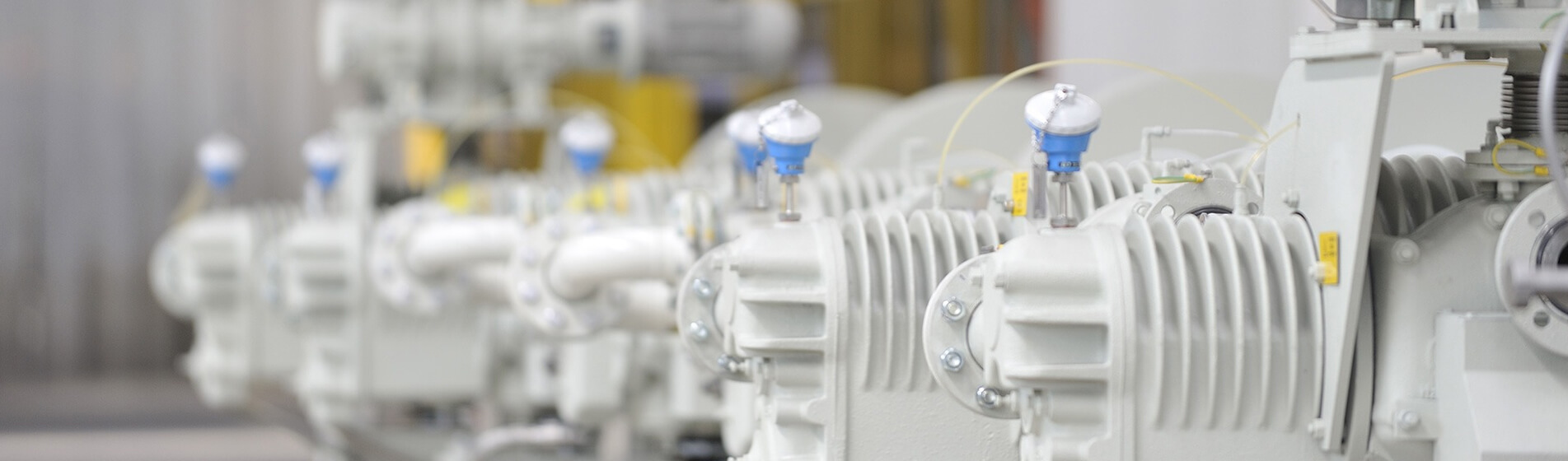News
Influence of turbine stage parameters of composite molecular pump on the performance of helium mass spectrometer leak detector Aug 02, 2022

Abstract: as an important component of the helium mass spectrometer leak detector, the geometric parameters of the composite molecular pump not only affect the pumping performance, but also affect the detection performance of the leak detector. Based on the basic theory of molecular pump pumping, this paper establishes the calculation model of composite molecular pump turbine stage. By changing the blade inclination, blade number, blade height and blade thickness, the pumping performance of composite molecular pump turbine stage on air and helium is calculated respectively, and the influence of composite molecular pump turbine stage parameters on the detection performance of helium mass spectrometer leak detector is studied. The results show that decreasing blade inclination, increasing the number and height of blades and increasing the thickness of blades are conducive to the improvement of the detection accuracy of helium mass spectrometer leak detector; Considering the pumping performance of the molecular pump and the detection accuracy of the helium mass spectrometer, it is optimal to select the blade inclination of 25 °, the number of turbine blades of 25 to 30, the blade height of 3mm, and the blade thickness of 0.6mm to 0.8mm.

Key words: compound molecular pump turbine stage blade parameters helium mass spectrometer leak detector detection accuracy

Helium mass spectrometer leak detector is widely used in the field of vacuum leak detection technology. It is a mass spectrometer specially used for vacuum leak detection with helium as the leakage gas. It is widely used in aerospace, electric power, petroleum, environmental protection and other fields [1-4]. Compared with other helium mass spectrometer leak detection equipment, the helium mass spectrometer has stable performance and high sensitivity [5-7]. As a vacuum acquisition device, the composite molecular pump is a key component of the helium mass spectrometer leak detector, and its pumping performance directly affects the detection performance of the leak detector . The domestic molecular pump started late, and there is still a gap with foreign products. The multi port composite molecular pump used in the helium mass spectrometry leak detection technology has not been developed in China, but relevant research has been carried out [9-10].

Helium mass spectrometer leak detector usually has two leak detection methods: forward flow detection and reverse flow detection, as shown in Figure 1 and Figure 2. During downstream leak detection, the tested piece is connected to the high vacuum side of the leak detector, indicating that after the leakage helium enters the vacuum system through the leak hole, part of it is pumped away, and the other part enters the mass spectrometry chamber and forms a leak signal after being caught. We hope that the compression ratio of the molecular pump to air is greater than that to helium as much as possible, so that the proportion of helium left in the mass spectrometry room is as large as possible, which is conducive to the ionization detection of helium, so as to improve the accuracy of the leak detector. During countercurrent leak detection, the tested piece is connected to the low vacuum side of the leak detector, and the tested piece is isolated from the mass spectrometry chamber by the main pump. The leaked helium enters the mass spectrometry chamber against the direction of the vacuum pump. We also hope that the compression ratio of the molecular pump to the air is as large as possible, so that the proportion of helium flowing back to the mass spectrometry chamber is as large as possible. In this paper, the influence of molecular pump performance on the detection accuracy of helium mass spectrometer leak detector will be studied through the ratio of the compression ratio K1 of molecular pump to air and the compression ratio K2 of molecular pump to helium. The larger the ratio is, the larger the proportion of helium in the mass spectrometer chamber is, which is more conducive to leak detection.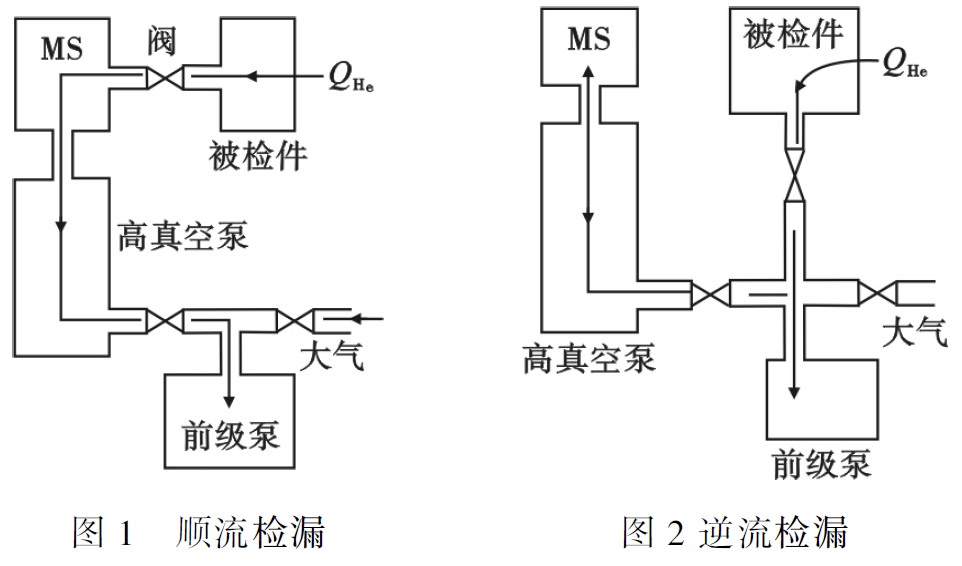The blade parameters of the turbine stage of the composite molecular pump directly affect the pumping performance of the molecular pump, and indirectly affect the detection performance of the helium mass spectrometer leak detector by changing the pumping performance. In this paper, the turbine stage of composite molecular pump for helium mass spectrometer leak detector is taken as the research object. Based on the extraction theory and calculation method of turbine molecular pump, a calculation model of turbine stage suitable for leak detection technology is established. By changing the inclination, number, height and thickness of turbine blades, the extraction performance of turbine stage is calculated separately, Combined with the actual use of helium mass spectrometer leak detector, the change of leak detection performance caused by the change of turbine blade parameters is studied. The influence of Turbine Blade Parameters on the detection performance of helium mass spectrometer leak detector and the optimal parameter value of turbine blade when considering the pumping performance of molecular pump and the detection accuracy of helium mass spectrometer are obtained, so that the composite molecular pump can be better applied to leak detection technology.

1 Calculation Model and method

Firstly, the calculation model of turbine stage of composite molecular pump for helium mass spectrometer leak detector is established, and the calculation method is determined.

1.1 calculation model of extraction performance of molecular pump turbine stage

The simplified calculation model is obtained by expanding the turbine stage single blade row, as shown in Figure 3. The thermal velocity of gas molecules conforms to Maxwell's velocity distribution law, and the adsorption and desorption on the blade row comply with the cosine law .The turbine blade divides the space into I and II. The pressure, molecular number density and temperature of gas molecules on both sides are expressed in P, Nd and t respectively. The blade thickness is t, the pitch chord ratio is a/b, and the channel port area is A1 and A2 respectively. The forward transmission probability is M12 and the reverse transmission probability is M21. Through derivation, the extraction performance of single-stage blade train can be obtained .

Maximum ho coefficient (the extraction coefficient representing the extraction efficiency):Maximum compression ratio: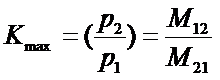Maximum pumping speed of single blade train: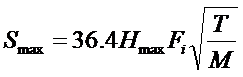Where: m is the molecular weight of the gas; FI is the sum of the end face areas of the i-th working wheel blade row channel.

It can be seen that the extraction characteristics of the turbine stage mainly depend on the transmission probability of gas molecules through the blade row M12 and M21. The turbine stage is usually composed of multi-stage moving blades and stationary blades in series. In the calculation process, the blade rows of the first stage and the second stage are calculated in series, and then the calculation results are calculated in series with the third stage. Finally, the overall forward and reverse transmission probability of the turbine stage is obtained .

The forward and reverse transmission probabilities of the first n-1 stage are defined as PN-1 and QN-1, the forward and reverse transmission probabilities of the nth stage single leaf train are defined as P1 and Q1, and the forward and reverse transmission probabilities of the first N stage leaf train are defined as PN and QN. Pn-1pn represents the unit flow gas molecules that can enter the turbine blade row channel and directly reach the exhaust side through the n-stage blade row; PN-1 (1-pN) refers to the gas molecules reflected from the nth stage blade row. The calculation model of combined blade row is shown1.2 calculation method

When the turbine stage of the composite molecular pump works in the molecular flow state, the Monte Carlo method can be used to calculate the pumping performance, and the more ideal calculation results can be obtained. When using Monte Carlo method to calculate the extraction performance of single blade row of turbine stage, the geometric parameters and linear velocity at the midpoint of the blade are used to calculate the extraction performance of the whole blade. This paper calls it engineering method. Such simplification will have a certain impact on the calculation accuracy. This paper adopts a new optimization algorithm of Monte Carlo method - integral mean method , which divides a single blade into s equal parts along the length direction, calculates the extraction characteristics according to the parameter values at each point, and then uses the integral method to calculate the average value of the extraction performance of the whole blade. This can greatly improve the calculation accuracy. The comparison between engineering method and integral mean method is shown in Figure 5.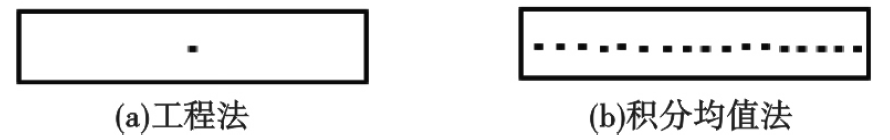The obtained single blade row extraction performance is interpolated, and the mathematical relationship between transmission probability and blade row parameters is established by data regression method, and then the transmission probability is directly calculated by calling the formula .

The calculation process of turbine stage combined blade row using MATLAB software is as follows: firstly, the basic parameters of turbine stage such as blade inclination, pitch chord ratio and speed ratio of each stage blade row are input according to the alternating sequence of moving and stationary blade rows; For each stage of blade row parameters input, the corresponding subroutine is automatically called to calculate the extraction performance once, and the blades are calculated in sections, and the average value is calculated by integration; In the calculation of transmission probability, the corresponding transmission probability formula of structural parameter matching is used. When the parameters of all turbine blade trains are input, the pumping speed and compression ratio of turbine stage combined blade trains are calculated by using the formula of combined blade trains. The program block diagram is shown in Figure 6.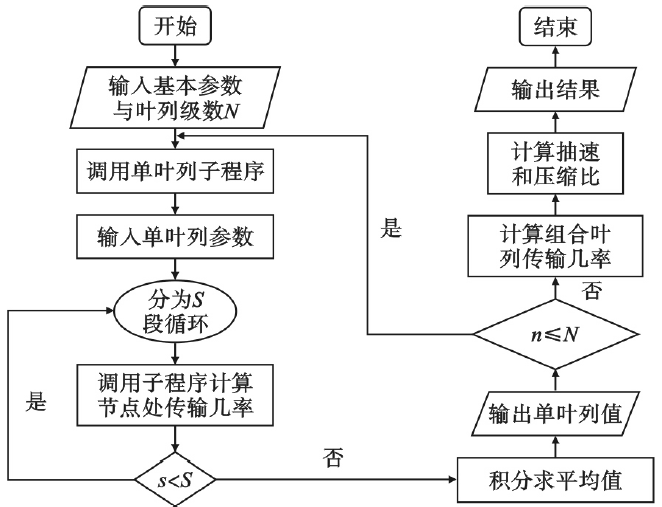According to the calculation model of the extraction performance of the turbine stage, the data of f-63 composite molecular pump are used to α、 The extraction performance of turbine blades with height h, thickness T and number of blades Z is calculated, and the influence of the above parameters on the extraction performance is analyzed; Aiming at the medium used in helium mass spectrometer leak detector, the pumping performance of composite molecular pump turbine stage for air and helium is calculated, compared and analyzed. The turbine speed is 72000r/min, the turbine outer diameter D, turbine inner diameter D, blade length L and other calculation parameters are shown in Table 1.

Table 1 turbine stage single blade parameters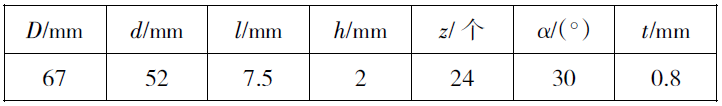2 Calculation and analysis

2.1 comparison of pumping speeds of different gases

When the composite molecular pump is applied to the helium mass spectrometer leak detector, it involves two working media: air and helium. Firstly, the difference of the pumping speed of the turbine blade for air and helium is analyzed.

The molecular weight m and the maximum ho coefficient Hmax in the pumping rate formula are different for different gas types. Figure 7 shows the relationship between the maximum ho coefficient Hmax and the speed ratio C under different blade inclination angles when the turbine blade row pitch chord ratio (a/b) is 1.2. Figure 8 shows blade inclination α= At 30 °, the relationship between the maximum Ho's coefficient Hmax and the velocity ratio C under different pitch chord ratios.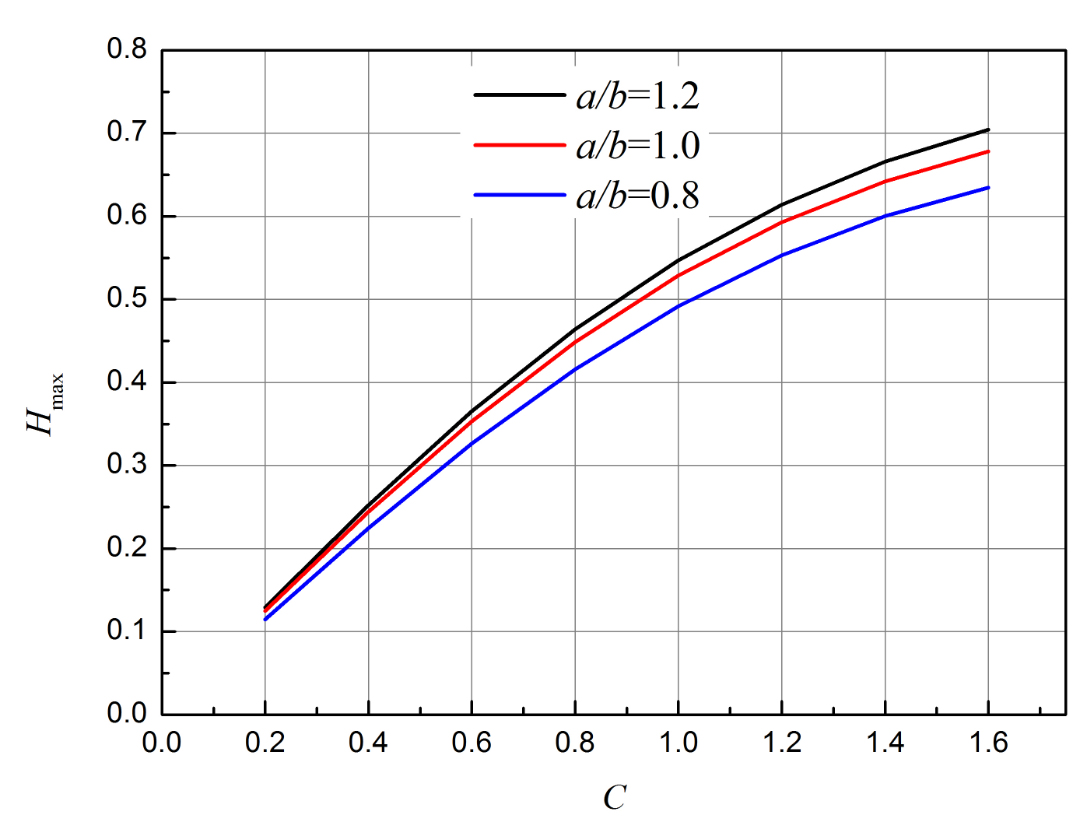It can be seen that within a certain range, the speed ratio C and the maximum ho coefficient Hmax show a linear change relationship, so for a given α And a/b, this linear relationship can be expressed as:Where: A is and α Constant related to a/b. Substituting equation (4) into pumping speed formula (3) can obtain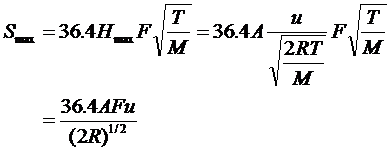It can be seen from formula (5) that when pipe resistance and impeller annular clearance are ignored, the pumping speed of turbine blade row for various gases is the same, and the maximum pumping speed when k=0 is independent of the type of pumped gas . When k ≠ 0, the working pumping speed of the pump increases slightly with the decrease of the molecular weight of pumped gas, and when the molecular weight of gas is different, the pipe resistance and gap backflow are different, which will also have a certain impact on the pumping speed. On this basis, only one gas will be selected for the calculation of the maximum pumping speed in the following study.

2.2 effect of blade inclination on the performance of helium mass spectrometer leak detector

Based on the single blade parameters of the turbine stage in Table 1, change the blade inclination, analyze its impact on the extraction performance, select the blade inclination of 10 °, 20 °, 30 °, 40 °, calculate the maximum compression ratio K1, K2 and pumping speed s of the turbine stage for air and helium, and the calculation results are shown in Figure 9.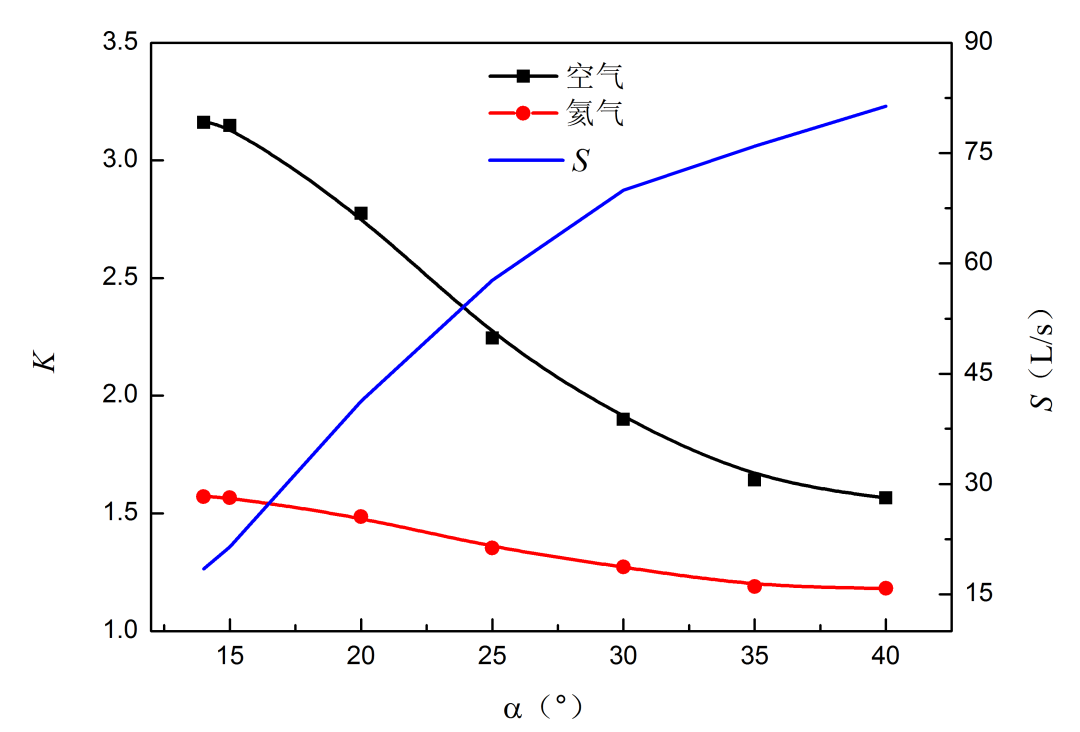It can be seen from Figure 9 that when other parameters are fixed, the extraction rate s increases with the increase of turbine blade inclination; The maximum compression ratio K decreases with the increase of blade inclination; The compression ratio of turbine blades to air molecules is greater than that of helium molecules.

When the composite molecular pump is used in the helium mass spectrometer leak detector, its compression ratio to air should be greater than that to helium as much as possible, which will be more conducive to the detection of leakage medium helium. The influence of blade inclination on the compression ratio of the two gases is further analyzed. Taking the compression ratio of turbine blade row to air and helium as the ratio, k1/k2 is obtained as a function of blade inclination α As shown in Figure 10.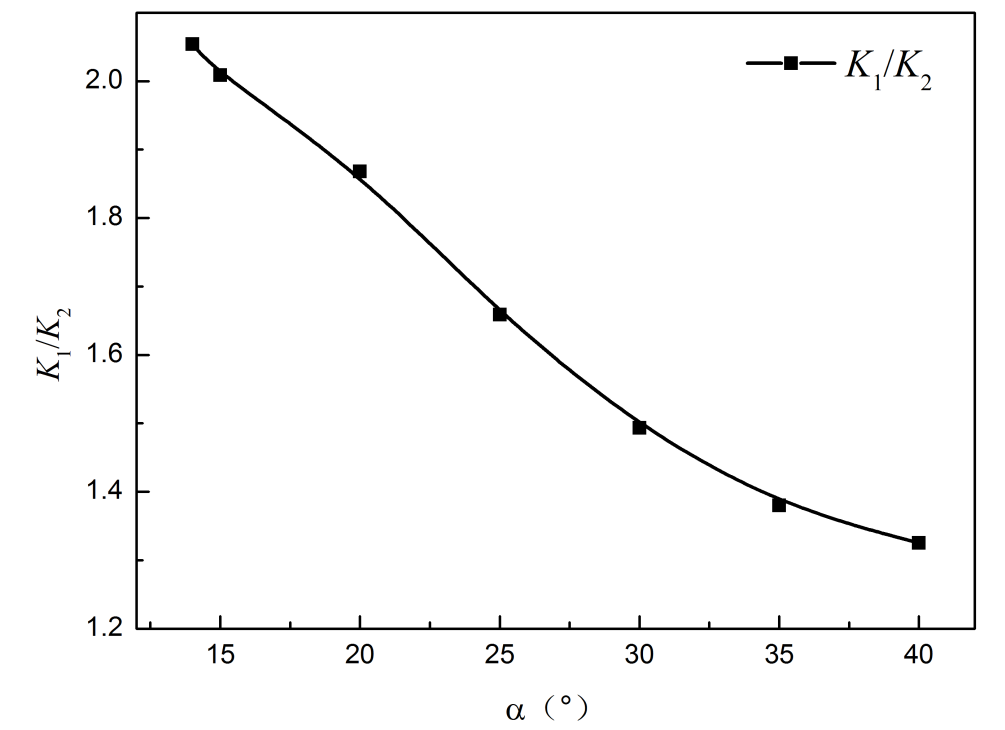It can be seen from Figure 10 that with the increase of the inclination angle, the ratio k1/k2 of the compression ratio of the turbine blade row to air and helium gradually decreases, indicating that the larger the inclination angle of the blade is, the more unfavorable it is to improve the detection sensitivity of the helium mass spectrometer, because the reduction of the compression ratio will cause the increase of the air return flow, the helium partial pressure in the mass spectrometer chamber will be reduced accordingly, and the detection pressure in the mass spectrometer chamber will be increased. When the pressure in the mass spectrometer chamber is greater than a certain value, it will no longer work, Therefore, the turbine stage of the composite molecular pump used as a helium mass spectrometer should be selected as small as possible, and the pumping rate should be considered. The compression ratio should be increased as much as possible on the premise of ensuring the pumping speed.

The ratio of the difference before and after the change of inclination angle to the parameter value before the change is defined as the change rate. The changes of the influence degree of the blade inclination angle on the compression ratio K1 of air, the compression ratio K2 of helium, the pumping speed s and k1/k2 are discussed. The results are shown in Table 2, in which negative values represent decrease and positive values represent increase.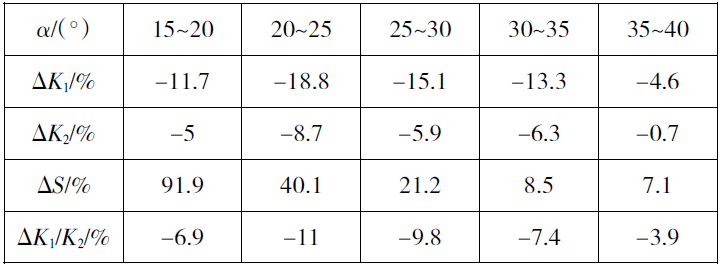It can be seen from the data in Table 2 that increasing the inclination angle will reduce the compression ratio and increase the pumping speed; With the increase of blade inclination, the change of compression ratio rises first and then decreases. The change rate of air compression ratio K1 is greater than that of helium compression ratio K2; The influence of dip angle on k1/k2 is the same. With the increase of dip angle, k1/k2 value decreases, and the change rises first and then decreases. From the above results, it is concluded that the increase of inclination angle can increase the pumping speed, but it will make the compression ratio of air drop faster, which is not conducive to the improvement of the detection accuracy of helium mass spectrometer leak detector.

Based on the above calculation results, when the blade inclination is about 25 °, the blade extraction speed can reach 57l/s, and k1/k2=1.65, which can obtain better extraction performance and higher leak detection accuracy.

2.3 effect of blade number on the performance of helium mass spectrometer leak detector

Based on the single blade parameters of the turbine stage in Table 1, the influence of the number of turbine blades on the pumping speed and compression ratio of the turbine stage is studied by changing the number of turbine blades. Taking 15 to 60 blades as the growth base, the maximum compression ratio of the turbine stage to air and helium and the pumping speed of air molecules are calculated respectively, and the ratio curve of the maximum compression ratio of air and helium is obtained, as shown in FIG. 11 and FIG. 12.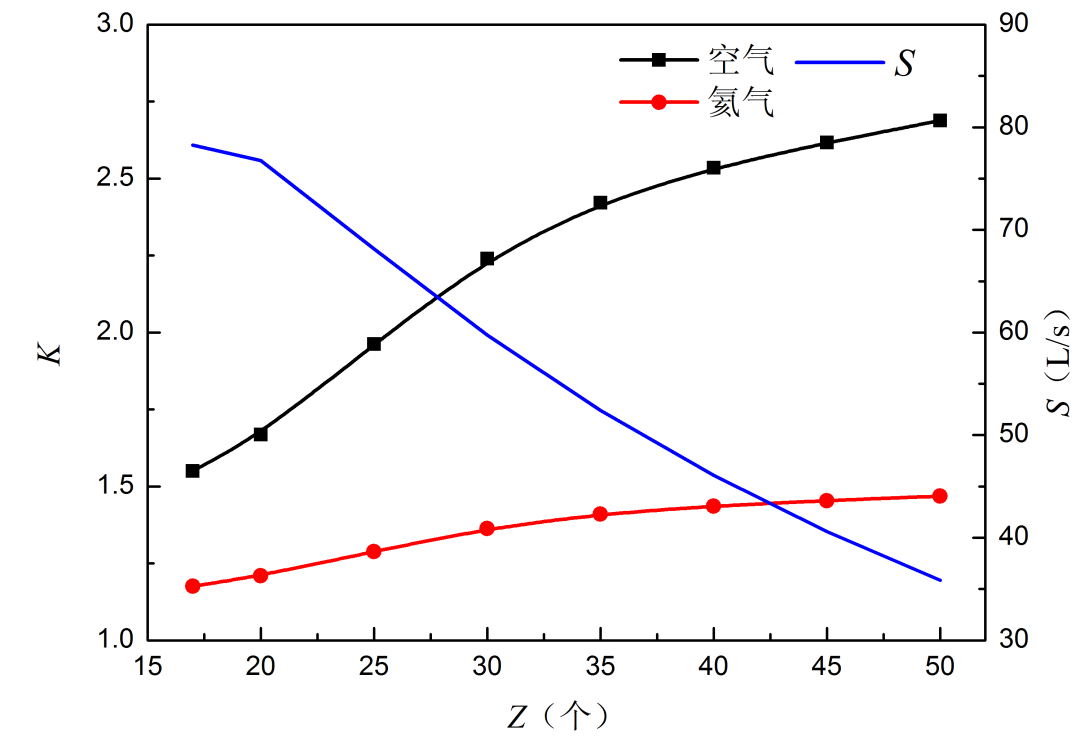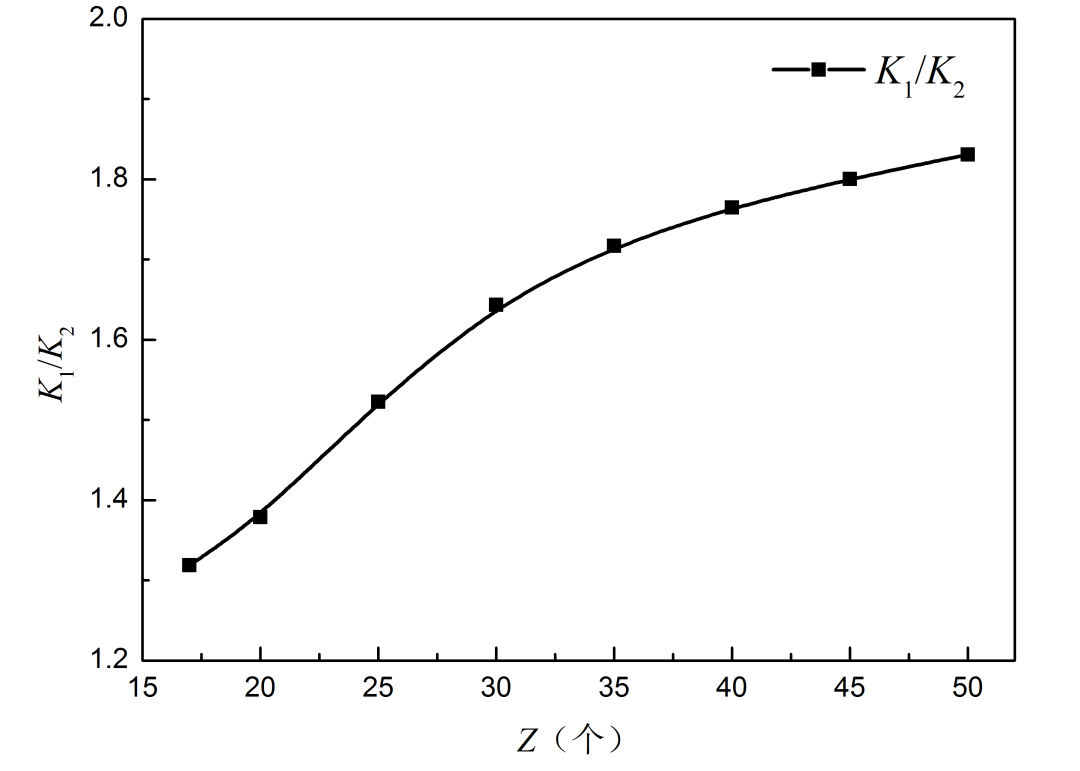It can be seen from Figure 11 that when other parameters remain unchanged, with the increase of the number of turbine blades, the pumping speed of the turbine stage gradually decreases and the compression ratio gradually increases; For air molecules, when the number of leaves gradually increases to 30, the compression ratio increases rapidly, and after the number of leaves exceeds 30, the compression ratio increases slowly; The compression ratio of helium molecules changes gently. The compression ratio increases slowly before the 35 stage blade row, and gradually stabilizes after the number of blades reaches 35. It can be seen from Figure 12 that the compression ratio of turbine blade row to air and helium is in direct proportion to the number of blades. With the increase of the number of blades, the compression ratio gradually increases, and the change process is first rapid and then slow. The more the number of blade rows, the higher the detection accuracy of helium mass spectrometer leak detector for leak rate.

Discuss the changes of the number of blades on the compression ratio K1 of air, the compression ratio K2 of helium, the pumping speed s and k1/k2. The results are shown in Table 3.It can be seen from the data in Table 3: with the increase of the number of blades, the compression ratio increases and the pumping speed decreases; With the increase of the number of blades, the change rate of compression ratio decreases, and the change rate of air compression ratio K1 is greater than that of helium compression ratio K2; With the increase of the number of leaves, k1/k2 value increased and the change rate decreased. From the above results, it is concluded that increasing the number of blades will make the air compression ratio rise faster, which is more conducive to the improvement of the detection accuracy of the helium mass spectrometer leak detector.

To sum up, according to the variation law of pumping speed and compression ratio with the number of blades, when the number of turbine blades is between 25 and 30, k1/k2 is between 1.52 and 1.64, the turbine blade row can obtain ideal pumping performance, and the helium mass spectrometer leak detector can obtain high detection performance.

2.4 effect of blade height on the performance of helium mass spectrometer leak detector

Based on the single blade parameters of the turbine stage in Table 1, the influence of changing the height of the blade on the extraction performance of the turbine blade row is analyzed. The blade height of 1.3mm to 8mm is selected, and the maximum compression ratio of the turbine blade row to air and helium and the pumping speed of the air are calculated for 9 different blade heights respectively. Then the ratio of the maximum compression ratio of air and helium is obtained, as shown in Figure 13 and Figure 14.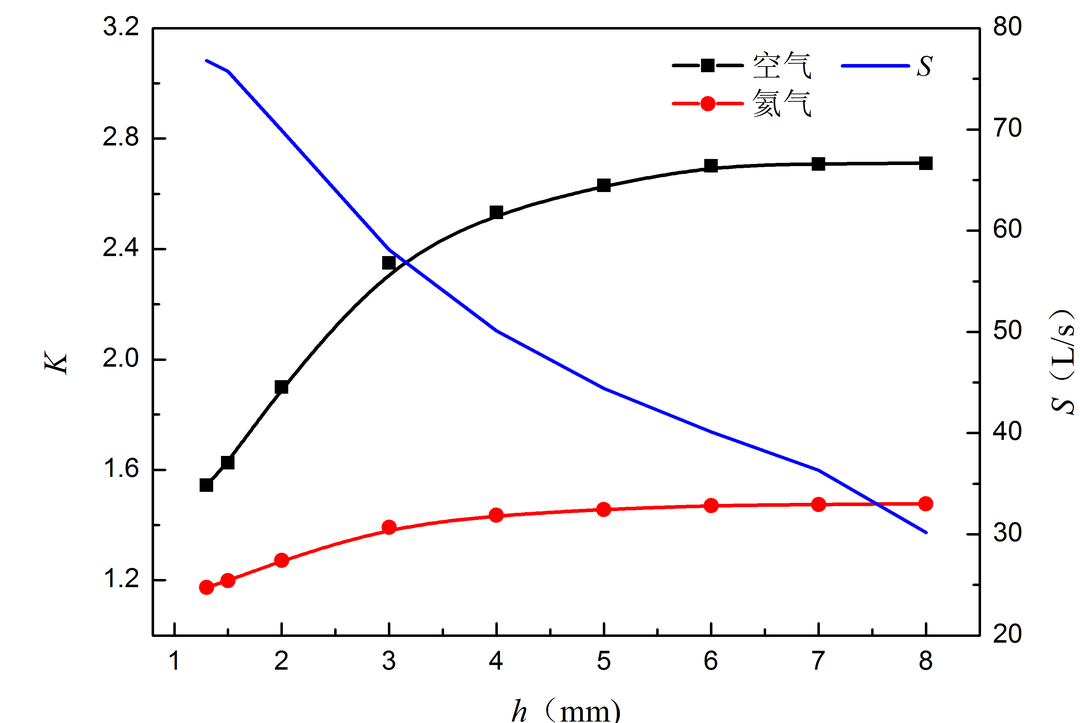As shown in Figure 13, when other parameters are fixed, the blade height is negatively correlated with the extraction rate of the turbine blade row and positively correlated with the maximum compression ratio; For air molecules, the compression ratio of turbine blade row first rises sharply until the blade height reaches 3mm, and then changes to a slow rise; For helium molecules, the compression ratio of turbine blade row rises gently, and rises slightly before the blade height reaches 3mm, and then tends to stabilize.

It can be seen from Figure 14 that the ratio of the compression ratio of turbine blade row to air and helium gradually increases with the increase of blade height. When the blade height is less than 3mm, it changes rapidly, and then the curve becomes flat.

The influence of blade height on air compression ratio K1, helium compression ratio K2, pumping speed s and k1/k2 is discussed. The results are shown in Table 4.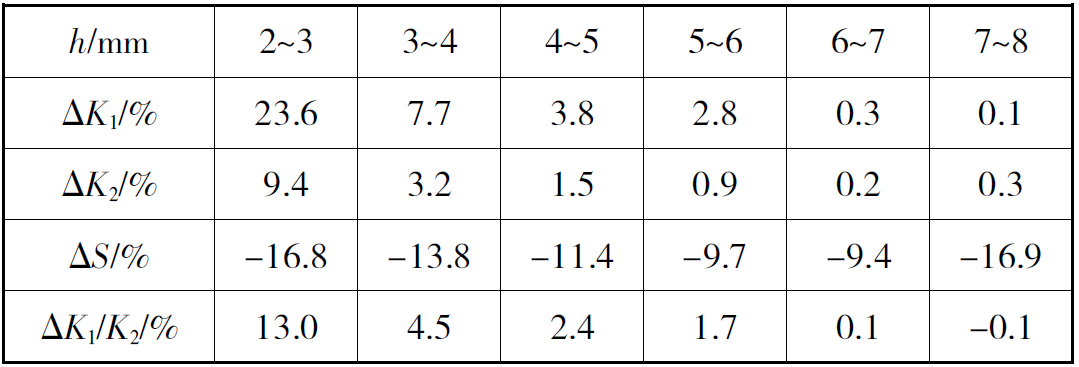It can be seen from the data in Table 4: with the increase of blade height, the compression ratio increases and the pumping speed decreases; With the increase of blade height, the change rate of compression ratio decreases, and the change rate of air compression ratio K1 is generally greater than that of helium compression ratio K2; With the increase of blade height, k1/k2 value increases and the change rate decreases. From the above results, it is concluded that increasing the blade height will make the air compression ratio rise faster, which is more conducive to the improvement of the detection accuracy of the helium mass spectrometer leak detector, but the pumping speed will be reduced.

According to the above calculation results, when the blade height is about 3mm, the k1/k2 value is 1.69, which can not only ensure the air extraction performance, but also obtain a large compression ratio to ensure the detection accuracy of the leak detector.

2.4 effect of blade thickness on the performance of helium mass spectrometer leak detector

Based on the single blade parameters of turbine stage in Table 1, the influence of blade thickness on the pumping speed and compression ratio of turbine blade row is analyzed. The blade thickness is selected as 0.1mm to 1mm, and the maximum compression ratio of turbine blade row to air and helium and the pumping speed of air under 10 blade thicknesses are calculated respectively. Then the ratio of the maximum compression ratio of air and helium is obtained, as shown in Figure 15 and Figure 16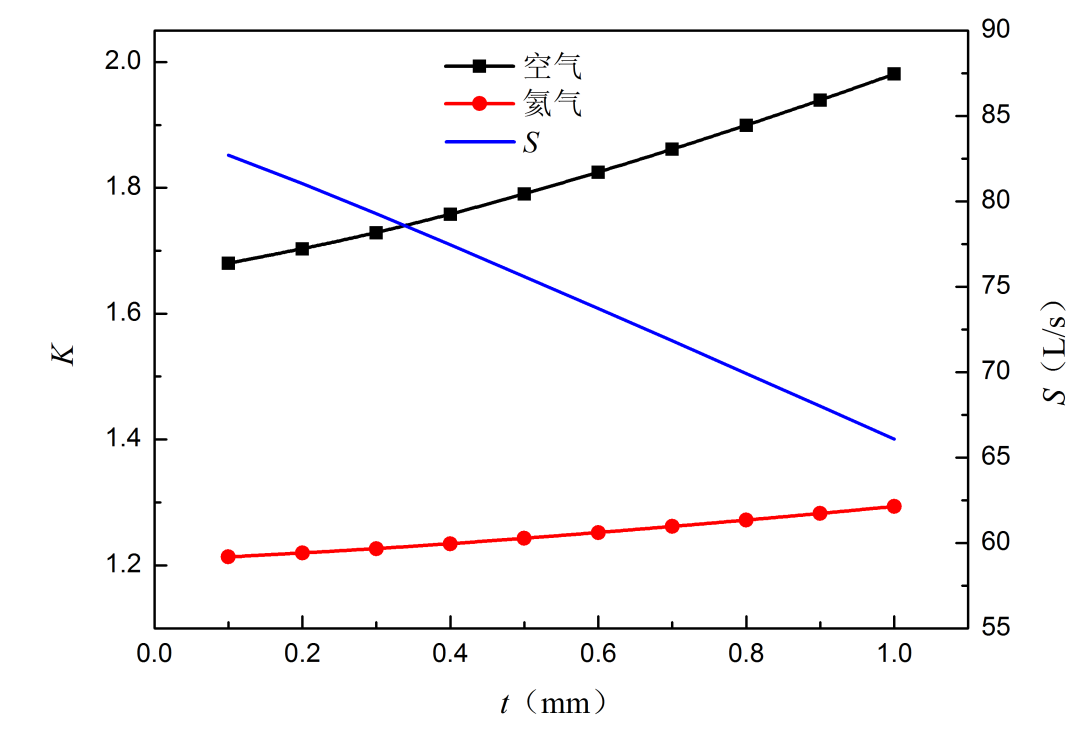It can be seen from Figure 15 that when other parameters are fixed, the pumping speed of turbine blade row gradually decreases with the increase of blade thickness, and the compression ratio of turbine blade row slowly increases with the increase of blade thickness. The thickness of the blade mainly affects the effective extraction area of the turbine stage. The thinner the blade, the larger the effective extraction area, the greater the pumping speed. The main function of the turbine stage is to improve the pumping speed of the composite molecular pump. Therefore, on the premise of ensuring the strength of the turbine blade, the thickness of the blade should be minimized [17-19].

It can be seen from Figure 16 that with the increase of blade thickness, the ratio of the compression ratio of air and helium continues to increase and changes smoothly. The influence of blade thickness on air compression ratio K1, helium compression ratio K2, pumping speed s and k1/k2 is discussed. The results are shown in Table 5.It can be seen from table 5 that with the increase of blade thickness, the compression ratio increases and the pumping speed decreases; The blade thickness has little effect on the air compression ratio K1, and the maximum change rate is 2.09%; The blade thickness has less influence on the compression ratio of helium, and the maximum is only 0.86%; With the increase of blade thickness, the change rate of compression ratio increases, and the change rate of air compression ratio K1 is greater than that of helium compression ratio K2; With the increase of blade thickness, k1/k2 value and change rate increase, and the maximum change rate is 2.83%. From the above results, it is concluded that increasing the thickness of the blade will make the air compression ratio rise faster, which is more conducive to the improvement of the detection accuracy of the helium mass spectrometer leak detector, but the pumping speed will be reduced; Blade thickness has little effect on the above parameters.

According to the above calculation results, when the blade thickness is 0.6mm to 0.8mm, the extraction performance and detection performance are better.

3 conclusion

In this paper, the extraction performance of the turbine stage single blade train of the compound molecular pump under the conditions of different blade inclination, blade number, blade height and blade thickness is calculated by using the integral mean method in the molecular flow pattern. The influence of the above Blade Parameters on the extraction performance is studied and discussed, and the following conclusions are obtained:

❶ with the increase of blade inclination, the compression ratio of the turbine stage of the composite molecular pump decreases and the pumping speed increases; The larger the blade angle is, the smaller the influence on the pumping speed and compression ratio is; In the process of increasing blade inclination, the compression ratio of turbine stage to air decreases faster than that of helium. Increasing blade inclination is not conducive to the improvement of detection accuracy of helium mass spectrometer leak detector.

❷ with the increase of the number of blades, the compression ratio of the turbine stage of the composite molecular pump increases and the pumping speed decreases; The larger the blade angle is, the smaller the influence on the compression ratio is, and the influence on the pumping speed is basically unchanged; In the process of increasing the number of blades, the compression ratio of turbine stage to air rises faster than that of helium. Increasing the number of blades is conducive to improving the detection accuracy of helium mass spectrometer leak detector.

❸ with the increase of blade height, the compression ratio of the turbine stage of the composite molecular pump increases and the pumping speed decreases; The higher the blade height is, the smaller the influence on the compression ratio is, and the influence on the pumping speed fluctuates little; In the process of increasing blade height, the compression ratio of turbine stage to air rises faster than that of helium. Increasing blade height is conducive to improving the detection accuracy of heli